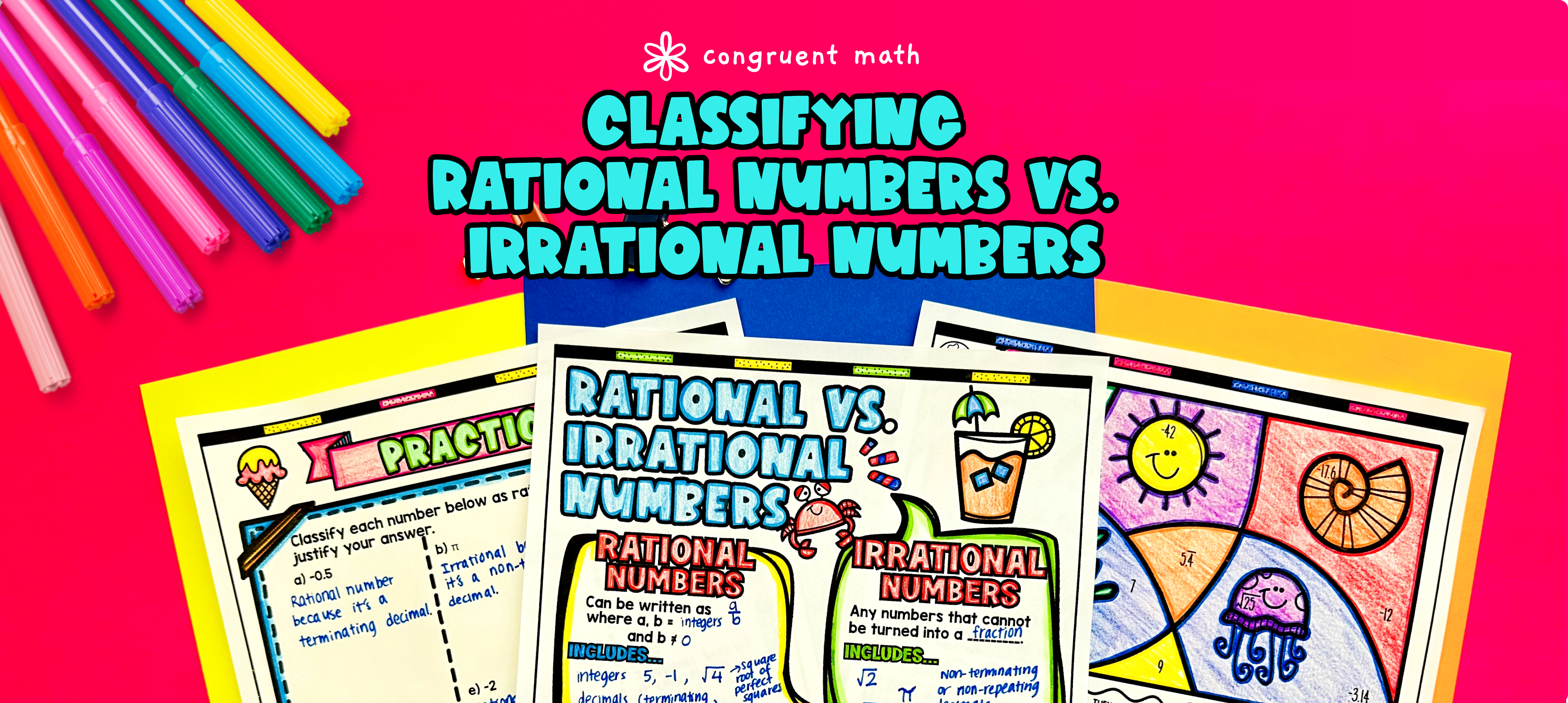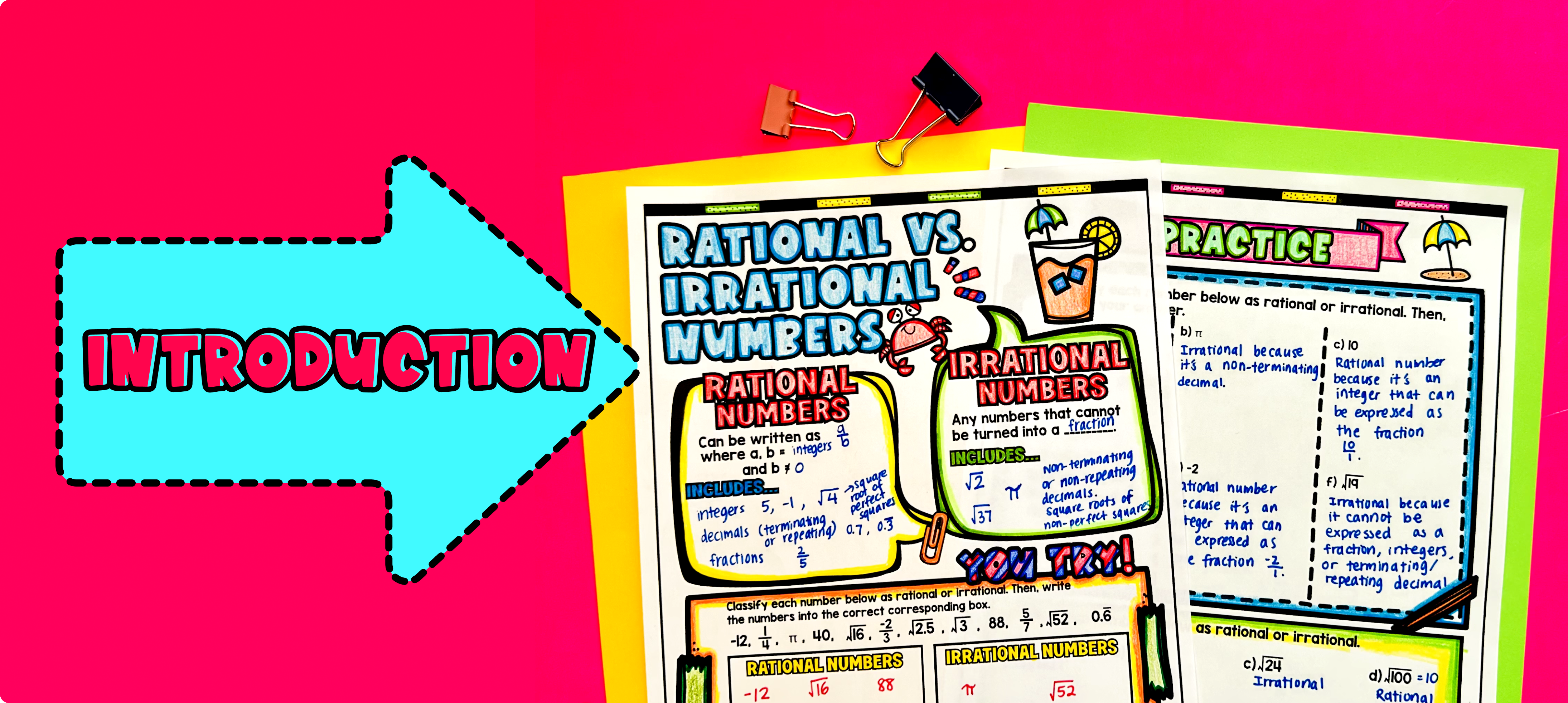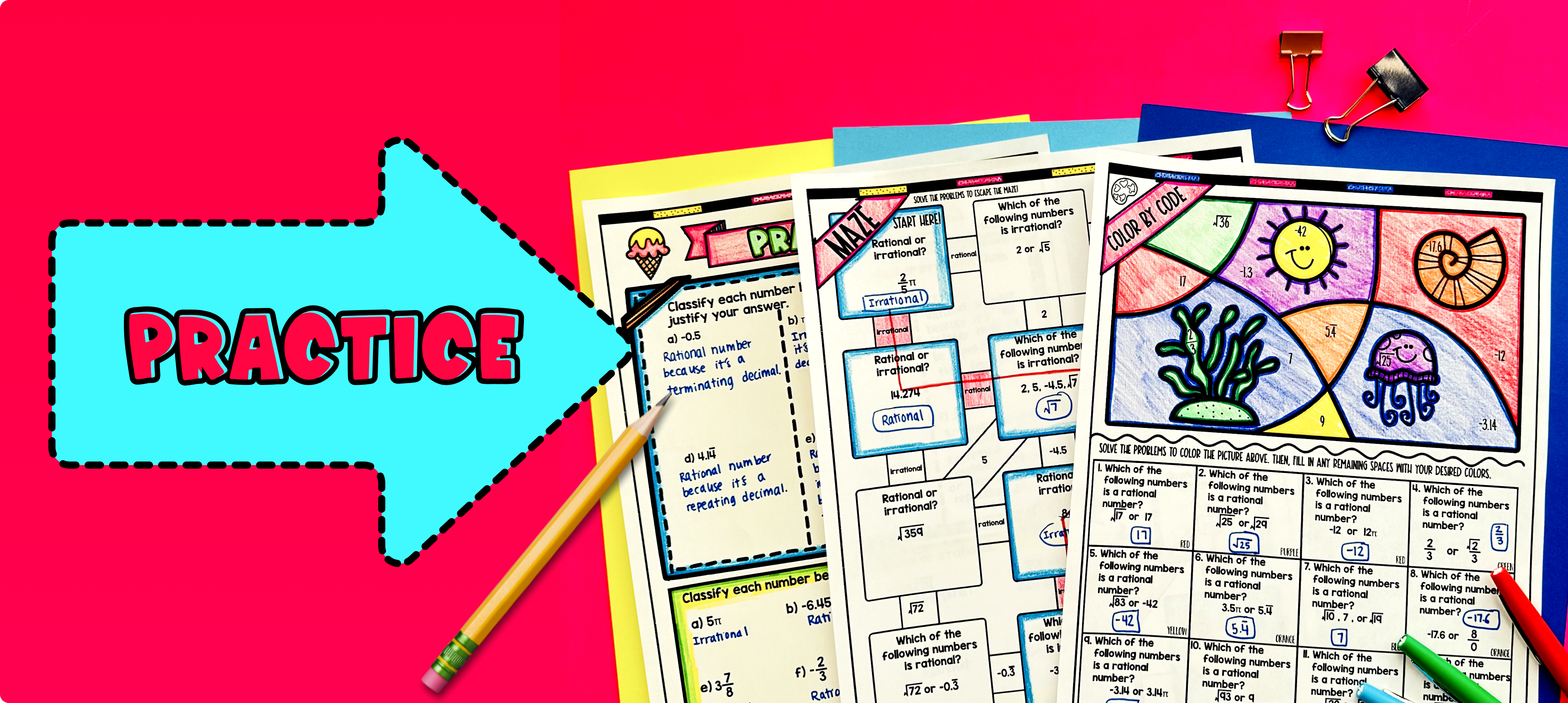# Classifying Rational and Irrational Numbers Lesson PlanPin This

## Overview

Ever wondered how to teach classifying rational and irrational numbers in an engaging way to your eighth-grade students?

In this lesson plan, students will learn about the different types of rational and irrational numbers and their real-life applications. Through artistic and interactive guided notes, checks for understanding, practice activities including a doodle & color by number activity, and a maze worksheet, students will gain a comprehensive understanding of classifying rational and irrational numbers.

The lesson culminates with a real-life example that explores how this concept is applied to staircase design. Students will read and write about this application, connecting their learning to the real world.

## Get the Lesson Materials\$4.25

## Learning Objectives

After this lesson, students will be able to:

• Classify rational numbers as integers, terminating decimals, or repeating decimals

• Classify irrational numbers as non-terminating/non-repeating decimals

• Explain the difference between rational and irrational numbers

• Apply the concept of classifying rational and irrational numbers to a real-life math application, specifically in the context of staircase design.

## Prerequisites

Before this lesson, students should be familiar with:

• The concept of rational numbers and their properties

• Understanding of the real number system

• Knowledge of integers and their properties

• Basic understanding of fractions, decimals, and their operations

## Key Vocabulary

• Rational Numbers

• Irrational Numbers

• Terminating Decimals

• Repeating Decimals

## Procedure

### IntroductionPin This

As a hook, ask students why classifying rational and irrational numbers is important in real life. You can use the question provided on the last page of the guided notes, such as "Why is it important to classify numbers as rational or irrational when designing a staircase?" This will engage students and get them thinking about the practical applications of classifying numbers.

Use the guided notes to introduce the topic of classifying rational and irrational numbers. Walk through the key points of the topic, including the different types of rational numbers (integers, terminating/repeating decimals, fractions) and irrational numbers in the real number system. Explain the significance of each type of number and how they can be identified.

Refer to the FAQ section below the guided notes for a walk through on how to introduce the topic and respond to common student questions. The FAQ section can provide additional guidance and support in teaching the concept effectively.

Throughout the introduction, be attentive to the needs of your students. If there is a wide range of proficiency levels in the class, consider pulling out students for reteaching while allowing more advanced students to begin working on the practice exercises. This will ensure that all students receive the appropriate level of support and challenge during the lesson.

### PracticePin This

Have students practice classifying rational and irrational numbers using the practice worksheet provided in the resource. Walk around the classroom to answer any student questions and provide support as needed.

Fast finishers can dive into the maze activity or the color by code activity for extra practice. You can assign one or both of these activities as homework for the remainder of the class.

### Real-Life ApplicationPin This

Bring the class back together, and introduce the concept of applying the classification of rational and irrational numbers to staircase design. Explain to the students that engineers and architects use rational numbers, such as fractions and terminating decimals, to design stairs that are safe and easy to climb. They need to consider the height of each step, as well as the total height of the stairs.

Use the following questions to facilitate a discussion with the students:

• How might architects and engineers use rational numbers in the design of stairs?

• What characteristics of rational numbers make them suitable for this application?

Encourage students to think about the different factors that could influence the design of stairs, such as building codes and safety regulations. Discuss how using rational numbers allows engineers to design stairs that meet these requirements.

Refer to the FAQ for more ideas on how to teach real-life applications of classifying rational and irrational numbers.